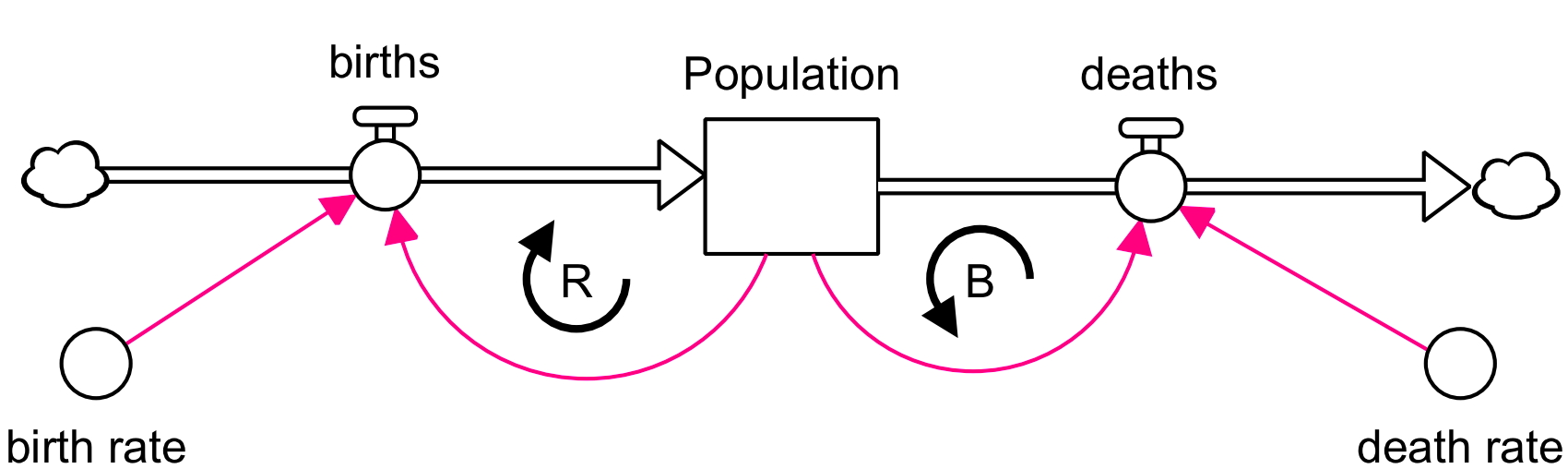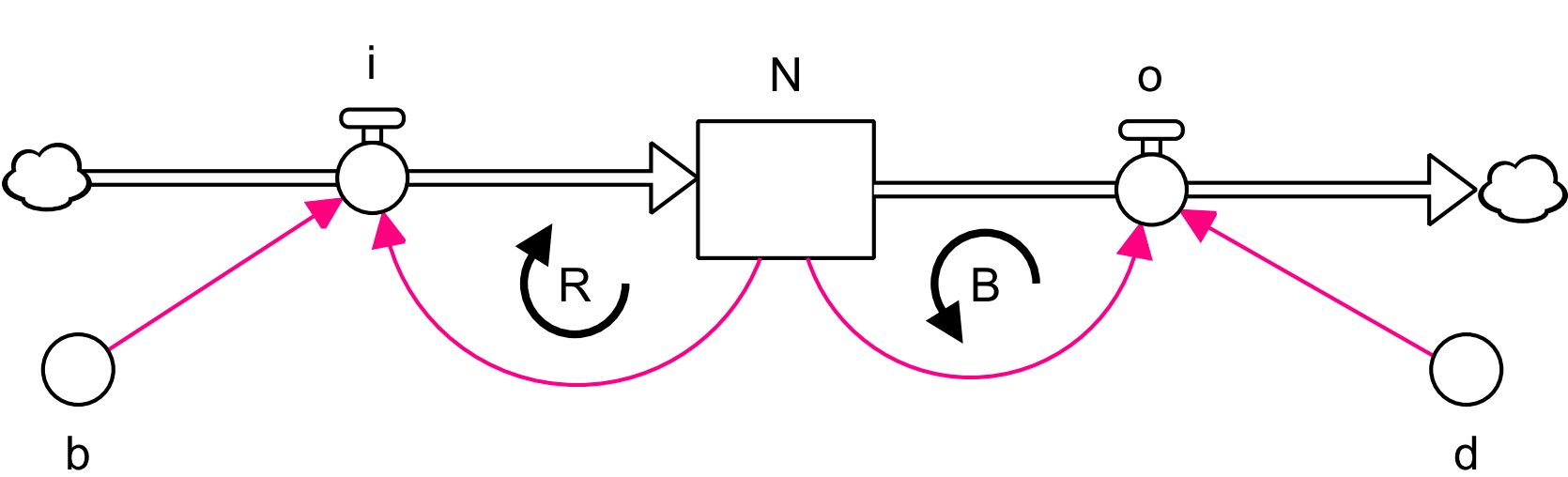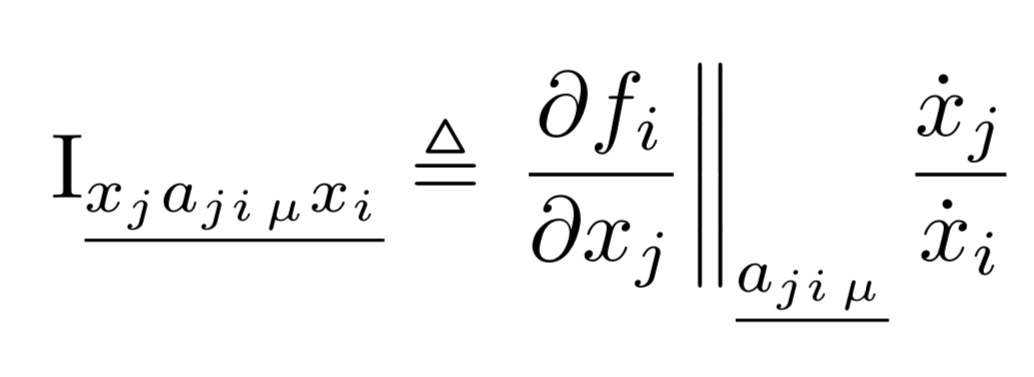# Loop Impact Notation

The use of the concept of impact to measure the effect of one stock on another, as found in feedback loops, requires system dynamics models to be written in symbolic notation.

A system dynamics model is more than a set of differential equations, which computes stock values at a given time from stock values at a previous time. The system dynamics model also records how each stock affects other stocks, i.e. the model contains all the pathways of cause and effect of the assumptions. Thus, a system dynamics model in analytic form needs more than a symbolic differential equation notation; it also requires notation to describe pathways between stocks.

For example, consider a population model with births and deaths:There are two links from the stock population to itself. Thus a symbolic representation of this model should capture these two links. Re-write the SD diagram with  symbols:where N = Population, i = births (inflow), o = deaths (outflow), b = birth rate and d = death rate. The SD model can now be expressed in purely symbolic notation:The underline subscripts indicate the pathway of influence of population N. This way the two forces in the model can be identified, along with their associated feedback loops, and their impacts calculated. If the pathway labels are removed, the equation reduces to the usual differential equation.

This pathway notation does mean that the general form of a system dynamics model can look a bit daunting:as does the general formula for force/loop impact:This notation is in the paper Hayward & Roach 2017. To help people understand this notation, I have put together a worked example relating these formulae to a specific model:

It assumes a basic knowledge of differential equation notation.